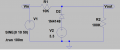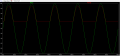# Understanding of positive voltage clipper with reverse diode - Help needed

#### mishra87

Joined Jan 17, 2016
958
Hi Guys,

I wanted to understand below circuit.
Could anybody explain the working of it and real time application of this circuit ?
Vout = Vbais - Vf

Forward condition (conduction mode) ?
Reverse conduction Mode (OFF mode) ?Last edited:

#### crutschow

Joined Mar 14, 2008
29,520
Under what condition does a diode conduct current, and under what condition does a diode block current.

Where do you think a voltage clipper circuit would be used?

#### mishra87

Joined Jan 17, 2016
958
Under what condition does a diode conduct current, and under what condition does a diode block current.

Where do you think a voltage clipper circuit would be used?

F.B - diode anode is more positive -: current flows
R.B. - Diode cathode is more positive-: block the current

But here +half cycle of sine wave diode cathode is more positive so it will block
Vout = 10x(100/110) = 9.09V
-half cycle of sine wave diode anode is more positive so it will conduct and
Vout = vbias -0.7 = 3.3-0.7= 2.6V

So why always 2.6V when it is conducting ?

So many applications of voltage clipper circuit but one is to protect voltage spikes or overvoltage .

I don't understand the application of this particularl circuit.

Regards

#### Wolframore

Joined Jan 21, 2019
2,533
Where did you find this example?

#### Wolframore

Joined Jan 21, 2019
2,533
There are all sorts of uses for diodes, that looks a lot like text book examples to show you how they work and what DC bias does to them... not necessarily part of any useful circuit.

How it works is simple. Diode only conducts when it's forward bias. You can change the bias by adding in this case a voltage source. It drops 0.7V.

#### crutschow

Joined Mar 14, 2008
29,520
So why always 2.6V when it is conducting ?
What does the V2 voltage minus the diode forward voltage drop equal?

#### ebeowulf17

Joined Aug 12, 2014
3,275
So why always 2.6V when it is conducting ?
I don't understand why you're asking the question above. You already answered it in your own words (quoted below.) The math seems to speak for itself. If you understand the math, what question is still troubling you?
-half cycle of sine wave diode anode is more positive so it will conduct and
Vout = vbias -0.7 = 3.3-0.7= 2.6V